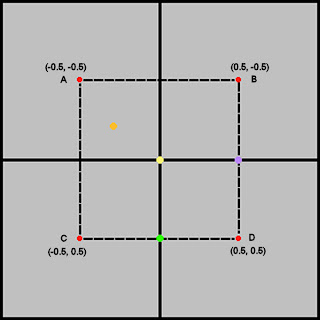## Wednesday, December 13, 2006

### Four Square Interpolation

This post is for those who like to think about math or enjoy puzzles. It's not meant to be overly tough, and who knows there might be several ways that it could be done. Anyway, this problem came up during work this week, and I thought it was somewhat interesting. Maybe some of you will find it interesting.Note that the y-axis might look flipped to you. This is because we are dealing with image coordinates, which are of the form (column, row).

Okay, so we've got 4 squares. Each square has a value associated with it: A, B, C, and D. The center intersection point is the origin (0, 0). Now, you see the orange point at some (x, y)? We want to know the interpolated value of (x, y) given the values A, B, C, and D for the squares. The range of (x, y) is bounded by the dotted square whose vertices are the center points of the 4 squares above. This is because if we were to get a point (x, y) that was outside of this bounds of the dotted square, then we would be dealing with a different set of four base squares.

So, let's take a few simple examples. Say we care about the yellow point at the origin. Then the proper interpolated value should be 0.25 * A + 0.25 * B + 0.25 * C + 0.25 * D. Say we are looking at the green dot at (0, 0.5). The proper interpolated value of that green dot should be 0.5 * C + 0.5 * D. Similarly, the proper interpolated value of the purple point should be 0.5 * B + 0.5 * D.

What we're really after is an interpolated value of the form: a * A + b * B + c * C + d * D. Simply put, how do you come up with the coefficients: a, b, c, and d.

So, give it some thought if you have the time and the interest. I'd like to hear what you come up with that works.